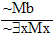Validity of Arguments involving Generalizations

Okay, we can show that some universal and existential arguments are invalid with our regular old pictures. For instance:

 Keith is hairy Mike is insane Ossie is hairy No-one is green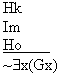is proved invalid byJeff is green Ossie is green Everything is greenis proved invalid by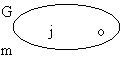But there are others that need the new stuff.
 Jeff is not a kangaroo A kangaroo existsis proved invalid by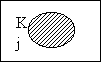Nigel is an elk A non-elk exists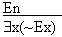is proved invalid by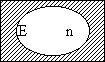Remember these statements?

Now read the following little arguments. Are they valid? I hope so, because they're intended to be arguments in which the statement above the line says exactly the same thing as the statement below it! Did I do it right?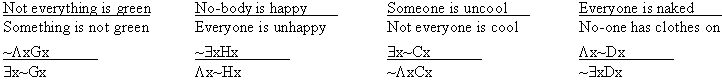Now compare each verbal argument with the symbolic argument immediately below it. They're meant to have the same logical structure and in fact, with the right scheme, they say exactly the same things.

What's going on here? Well, the fact is that certain general statements are logically equivalent to other general statements involving the opposite quantifier. Take a look at the following diagrams.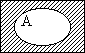Isx(Ax) true here? Is ~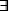x(~Ax) true here?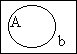Isx(Ax) false here? Is ~x(~Ax) false here?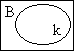Isx(Bx) true here? Is ~x(~Bx) true here?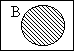Isx(Bx) false here? Is ~x(~Bx) false here?Is ~x(Bx) true here? Isx(~Bx) true here?Is ~x(Bx) false here? Isx~(Bx) false here?Is ~x(Cx) true here? Isx(~Cx) true here?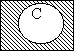Is ~x(Cx) false here? Isx(~Cx) false here?

Practice 12. Use your own paper or the answer sheet at Practice Sheet.

For each of the following arguments do TWO things. First, translate it into English based on the following scheme.

 Fx: x is fiendish Gx: x is a gryphon Hx: x is horrible Ix: x is insane Jx: x is a jumbuck Kx: x is kinky Lx: x is looney Mx: x is mad m: Merlin o: Ozymandias a: Ahuramazda b: Bob

Second, circle it if it's valid, cross it out if it's invalid.

 1.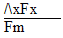2.3.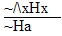4.5.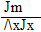6.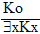7.8.